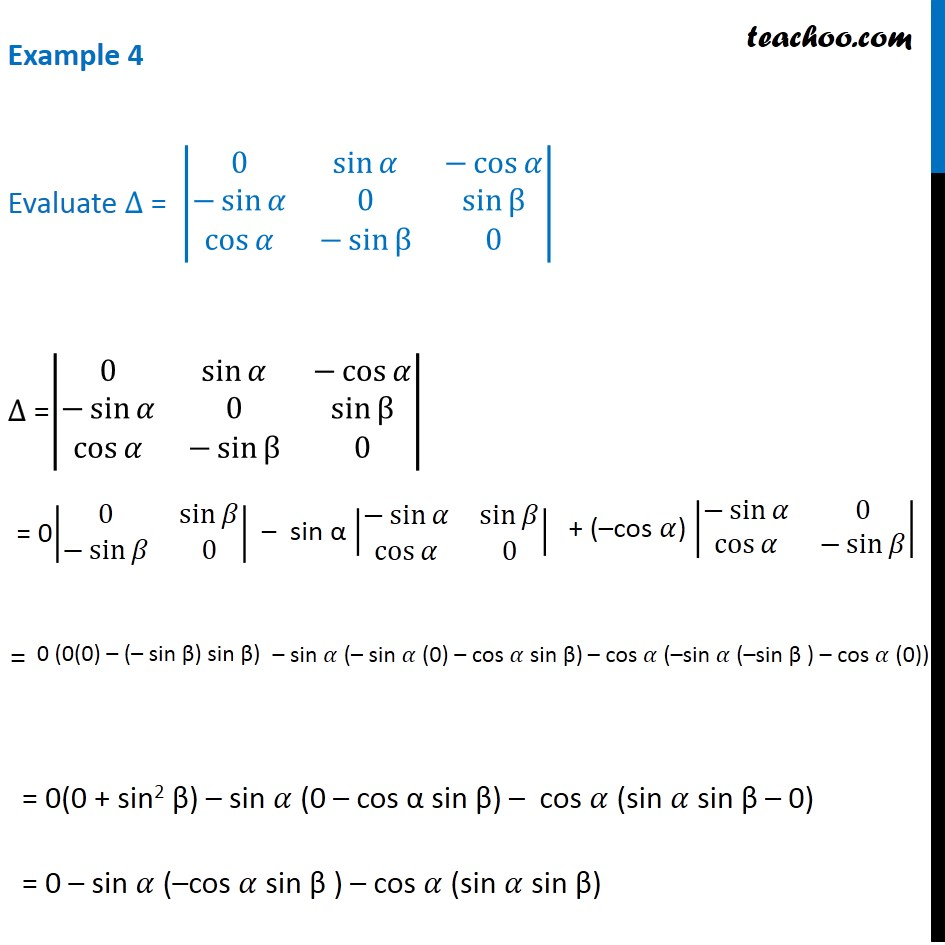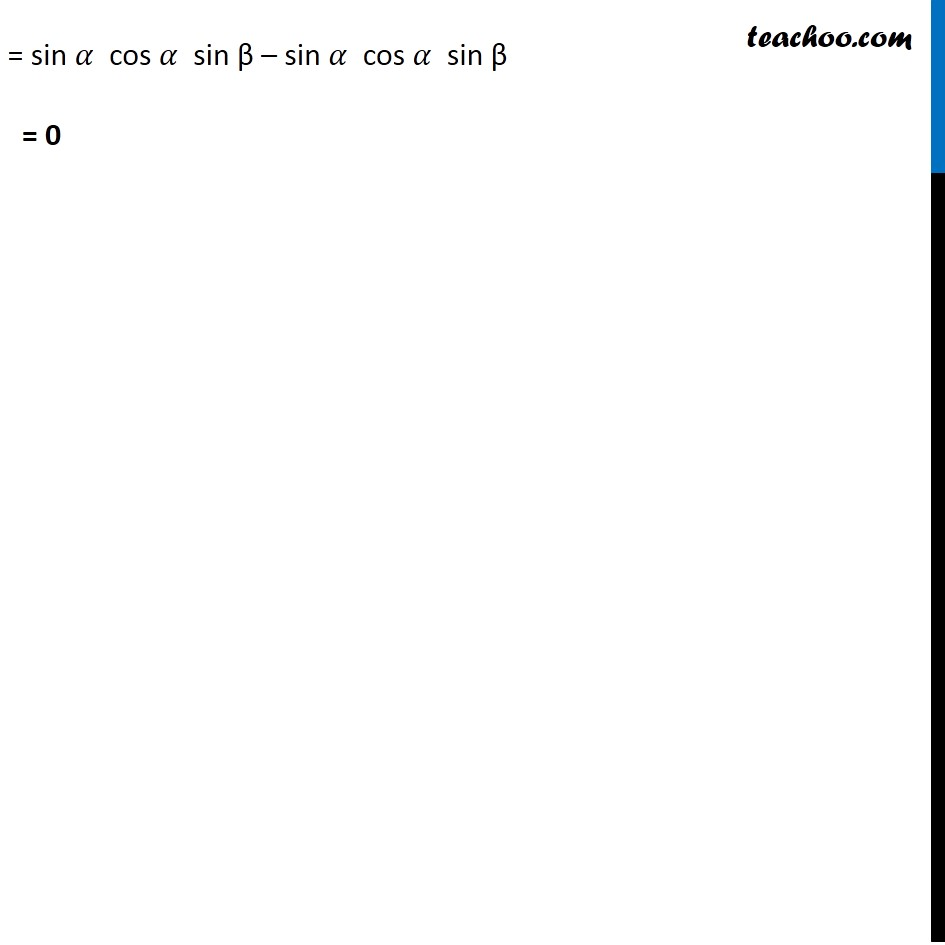Examples

Chapter 4 Class 12 Determinants
Serial order wiseLearn in your speed, with individual attention - Teachoo Maths 1-on-1 Class

### Transcript

Example 4 Evaluate ∆ = |■8(0&sin⁡𝛼&−cos⁡𝛼@−sin⁡𝛼&0&sin⁡β@cos⁡𝛼&−sin⁡β&0)| ∆ =|■8(0&sin⁡𝛼&−cos⁡𝛼@−sin⁡𝛼&0&sin⁡β@cos⁡𝛼&−sin⁡β&0)| = 0(0 + sin2 β) – sin 𝛼 (0 – cos α sin β) – cos 𝛼 (sin 𝛼 sin β – 0) = 0 – sin 𝛼 (–cos 𝛼 sin β ) – cos 𝛼 (sin 𝛼 sin β) = sin 𝛼 cos 𝛼 sin β – cos 𝛼 sin 𝛼 sin β = 0|■8(0&sin⁡𝛽@−sin⁡𝛽&0)| – sin α |■8(−sin⁡𝛼&sin⁡𝛽@cos⁡𝛼&0)| + (–cos 𝛼) |■8(−sin⁡𝛼&[email protected]⁡𝛼&−sin⁡𝛽 )| 0 (0(0) – (– sin β) sin β) – sin 𝛼 (– sin 𝛼 (0) – cos 𝛼 sin β) – cos 𝛼 (–sin 𝛼 (–sin β ) – cos 𝛼 (0)) = sin 𝛼 cos 𝛼 sin β – sin 𝛼 cos 𝛼 sin β = 0